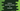# Example of python operator module lt## Example of python operator module lt:

Python operator modules provides different methods for standard operations. lt is used for less than. lt(first, second) is equivalent to first < second. In this post, I will show you how to use lt method of operator module with example.

### How to use operator module :

To use any method of operator module, we need to import it using the below line:

``import operator``

Then, we can use any of its method like lt as like below:

``operator.lt(first, second)``

The return value of lt is boolean and it returns True if the first value is less than the second value. Else, it returns False.

### Example of lt:

Below example shows how to use lt :

``````import operator

print(operator.lt(4,5))
print(operator.lt(10, 100))
print(operator.lt('3', '9'))
print(operator.lt('a', 'z'))
print(operator.lt('z', 'a'))``````

It will print:

``````True
True
True
True
False``````

### By taking user inputs:

We can also use lt by taking user inputs and compare the values using lt instead of < :

``````import operator

firstNumber = int(input('Enter the first number : '))
secondNumber = int(input('Enter the second number : '))

if(operator.lt(firstNumber, secondNumber)):
print('{} is less than {}'.format(firstNumber, secondNumber))
else:
print('{} is greater than {}'.format(firstNumber, secondNumber))``````

It will give outputs as like below: